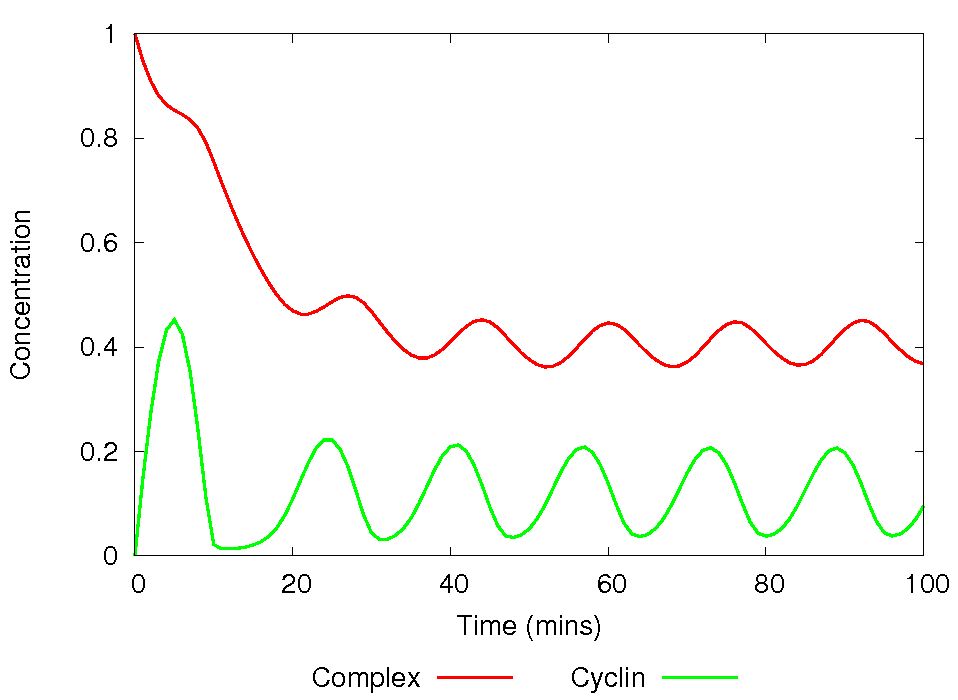## Gardner1998 - Cell Cycle GoldbeterModel Identifier
BIOMD0000000008
Short description
Gardner1998 - Cell Cycle Goldbeter

Mathematical modeling of cell division cycle (CDC) dynamics.

The SBML file has been generated by MathSBML 2.6.0.p960929 (Prerelease Version of 29-Sept-2006) 1-October-2006 15:36:36.076517.

This model is described in the article:

Gardner TS, Dolnik M, Collins JJ.
Proc. Natl. Acad. Sci. U.S.A. 1998 Nov; 95(24): 14190-14195

Abstract:

We demonstrate, by using mathematical modeling of cell division cycle (CDC) dynamics, a potential mechanism for precisely controlling the frequency of cell division and regulating the size of a dividing cell. Control of the cell cycle is achieved by artificially expressing a protein that reversibly binds and inactivates any one of the CDC proteins. In the simplest case, such as the checkpoint-free situation encountered in early amphibian embryos, the frequency of CDC oscillations can be increased or decreased by regulating the rate of synthesis, the binding rate, or the equilibrium constant of the binding protein. In a more complex model of cell division, where size-control checkpoints are included, we show that the same reversible binding reaction can alter the mean cell mass in a continuously dividing cell. Because this control scheme is general and requires only the expression of a single protein, it provides a practical means for tuning the characteristics of the cell cycle in vivo.

To the extent possible under law, all copyright and related or neighbouring rights to this encoded model have been dedicated to the public domain worldwide. Please refer to CC0 Public Domain Dedication for more information.

Format
SBML (L2V4)
Related Publication
• A theory for controlling cell cycle dynamics using a reversibly binding inhibitor.• Gardner TS, Dolnik M, Collins JJ
• Proceedings of the National Academy of Sciences of the United States of America , 11/ 1998 , Volume 95 , pages: 14190-14195 , PubMed ID: 9826676
• Center for BioDynamics and Department of Biomedical Engineering, Boston University, 44 Cummington Street, Boston, MA 02215, USA.
• We demonstrate, by using mathematical modeling of cell division cycle (CDC) dynamics, a potential mechanism for precisely controlling the frequency of cell division and regulating the size of a dividing cell. Control of the cell cycle is achieved by artificially expressing a protein that reversibly binds and inactivates any one of the CDC proteins. In the simplest case, such as the checkpoint-free situation encountered in early amphibian embryos, the frequency of CDC oscillations can be increased or decreased by regulating the rate of synthesis, the binding rate, or the equilibrium constant of the binding protein. In a more complex model of cell division, where size-control checkpoints are included, we show that the same reversible binding reaction can alter the mean cell mass in a continuously dividing cell. Because this control scheme is general and requires only the expression of a single protein, it provides a practical means for tuning the characteristics of the cell cycle in vivo.
Contributors
Nicolas Le Novère

isDerivedFrom
BioModels Database BIOMD0000000003
BioModels Database BIOMD0000000004
is
BioModels Database MODEL6614879888
BioModels Database BIOMD0000000008
isDescribedBy
PubMed 9826676
hasTaxon
Taxonomy Amphibia
isVersionOf
Gene Ontology mitotic cell cycle
isHomologTo

Curation status
Curated

Tags
Name Description Size Actions

### Model files

BIOMD0000000008_url.xml SBML L2V4 representation of Gardner1998 - Cell Cycle Goldbeter 27.94 KB Preview | Download

• Model originally submitted by : Nicolas Le Novère
• Submitted: Sep 13, 2005 1:36:20 PM
##### Revisions

(*) You might be seeing discontinuous revisions as only public revisions are displayed here. Any private revisionsof this model will only be shown to the submitter and their collaborators.

Legends
: Variable used inside SBML models

Species
Species Initial Concentration/Amount
C

IPR006670
0.0 mol
X

peptidase activity
0.0 mol
M

Cyclin-dependent kinase 1-A ; Cyclin-dependent kinase 1-B
0.0 mol
Y 1.0 mol
Z

IPR006670
1.0 mol
Reactions
Reactions Rate Parameters
vi

vi
vi=0.1
a1*C*Y

a1*C*Y
a1=0.05
alpha*d1*Z

alpha*d1*Z
alpha=0.1; d1=0.05
V3*(1+-1*X)*(K3+-1*X+1)^-1

V3*(1+-1*X)*(K3+-1*X+1)^-1
K3=0.2; V3 = NaN
V4*X*(K4+X)^-1

V4*X*(K4+X)^-1
K4=0.1; V4=0.1
M*V2*(K2+M)^-1

M*V2*(K2+M)^-1
K2=0.1; V2=0.25
a2*Z

a2*Z
a2=0.05
alpha*kd*Z

alpha*kd*Z
kd=0.02; alpha=0.1
vs

vs
vs=0.2
d1*Y

d1*Y
d1=0.05
C*k1*X*(C+K5)^-1

C*k1*X*(C+K5)^-1
k1=0.5; K5=0.02Curator's comment:
(added: 10 Aug 2009, 15:53:31, updated: 10 Aug 2009, 15:53:31)
Figure 4B of the reference publication is reproduced here. The model was simulated using Copasi v4.5 (Build 30)